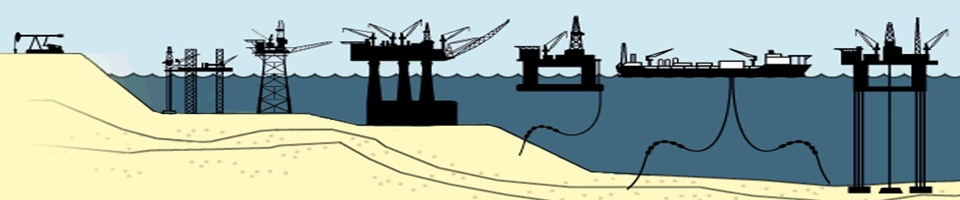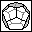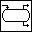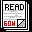####Gas-Oil-Water AppsHome  -  AGA-3  -  ISO-5167  -  Gas-Leak  -  Restriction Orifice  -  GOWProp  -  WatGas  -  GOSep  -  Contact

Easy to use engineering apps for the oil and gas industry - designed for practical field applications.
Apps have been tested and proven both on offshore and onshore oil and gas installations.

Designed for iPhone or iPad - available from the AppStore, or Excel macros (Excel 2007+).

Note that users must accept this disclaimer of warranty:
Each program is provided "as is" without warranty of any kind, either expressed or implied, including any warranty of merchantability or fitness for a particular purpose.  In no event shall the Author/Developer be held liable for any loss of profit, special, incidental, consequential, or other similar claims.

 Available AppsInfo Gas-Leak estimates gas-leak rates from piping based on restriction orifice calculations as per R.W. Miller's "Flow Measurement Handbook", assuming critical flow across the leak point. GasLeakCalc - DocumentationFree IPhone AppInfo AGA-3 calculates size, flowrate or pressure drop for gas orifice meters based on the American Gas Association AGA Report No. 3 (API Manual of Petroleum Measurement Standard, Chapter 14.3). AGA-3 - DocumentationAGA-3 in App-storeInfo ISO-5167 calculates size, flowrate or pressure drop for gas and liquid flow orifice meters based on International Standard ISO-5167-2:2003. ISO-5167 - DocumentationISO-5167 in App-storeInfo Restrict_Ori calculates size and flowrates for gas and liquid restriction orifice meters based on R.W. Miller's "Flow Measurement Handbook". Restriction Orifice - DocumentationRestrict_Ori in App-storeCurve-Fit uses regression analysis by method of Least Squares to fit an equation to a given data set.  Straight line curve-fitting is free, while other equations (10 available) require In-App purchase. Curve-Fit - DocumentationCurve-Fit in App-storeInfo GOW-Prop calculates physical properties (PVT) of Gases (Natural Gas, Air and Nitrogen) and Liquids (Oil, Water, MeOH, MEG, DEG and TEG) based on published correlations. Download Free Demo-versionInfo WatGas performs calculations for: - Hydrate Formation Conditions - Water Content of Natural Gases - MeOH/Glycol Inhibition Requirements - Solid CO2 Formation Conditions Download Trial-versionInfo GOSep performs flash calculations through two Oil/Gas separator stages optimize liquid recovery depending on pressure/temperature at each separator stage.  Free App. Download Free Excel AppFree Excel AppGOWDoc is the free documentation for the listed programs with correlations included (PDF-file). Download Free Documentation - PDF)Free Documentation - PDFFor more information, please e-mail questions/queries to: oilgasapp@gmail.com Home AGA-3 Orifice Calculations   Program AGA3  will size orifice plates for given design conditions, find pressure drop for a given flow, or flow for a given pressure drop. The standard (AGA-3 ( API-2530: 1991) is originally designed for gas orifices. In this program the standard is also used for liquid orifices. Gas calculations are performed for Natural Gas, Nitrogen and Air.  For liquid orifice calculations, the options are Crude Oil, Water, Methanol, Mono-ethylene glycol (MEG), Diethylene glycol (DEG) and Triethylene glycol (TEG). Input requirements are specific gas gravity, temperature and pressure for gas calculations. You can also give mole-fractions of N2, CO2 and H2S for sour gas calculations.  The AGA-8 equation is used for calculating Z-factor (compressibility factor) for natural gases, and the Redlich-Kwong equation of state for Nitrogen and Air. For oil-calculations you have to enter specific oil gravity, temperature and pressure. It is also recommended to give molecular weight of oil. For water-calculations the input requirements are salinity, temperature, and pressure.  It is assumed that all dissolved solids for water are expressed as equivalent sodium chloride concentration. The result will be an orifice specification sheet giving the necessary data for design of an orifice or evaluating an existing orifice.  The results will contain a few factors that you should know: The velocity of approach factor is defined as: Ev = 1/(Sqrt(1-Beta^4)) The flow coefficient, Alpha, is defined as: Alpha = Ev * Cd and orifice to pipe diameter ratio is given as: Beta = OD/PID The basic flow equation is: Qm = C*E*Eps*Pi/4*OD^2*Sqrt(2*dP*Roh) where Qm = Mass flow rate (kg/s) C = Discharge coefficient = Alpha/E E = Velocity of approach factor = 1/(Sqrt(1-Beta^4)) Eps = Expansion factor due to pressure drop Pi = 3.14159 OD = Orifice diameter at actual flowing conditions dP = Differential pressure across orifice Roh = Density of flowing fluid measured at upstream tap API/ ANSI-2530 - 1991 (AGA Report No. 3) (AGA) The basic flow equation is: Qv = Fn*(Fc+Fsl)*Y1*Fpb*Ftb*Ftf*Fgr*Fpv*Sqrt(Pf1*hw)   where Fn = Numeric conversion factor Cd = Discharge coefficient = (Fc + Fsl) Fc = Orifice calculation factor Fsl= Slope factor Y1 = Expansion factor based on upstream tap Fpb= Pressure base factor, set to 1.0 (14.73 psia) Ftb= Temperature base factor, set to 1.0 (60 deg F) Ftf= Flowing temperature factor The velocity of approach factor is defined as: Ev = 1/(Sqrt(1-Beta^4)) The flow coefficient, Alpha, is defined as: Alpha = Ev * Cd and orifice to pipe diameter ratio is given as: Beta = OD/PID Fgr= Specific gravity factor Fpv= Super-compressibility factor Pf1= Absolute flowing pressure based on upstream tap hw = Orifice differential pressure, in H2O at 60 deg F The above equation is often simplified to: Qv = C' * Sqrt(Pf1*hw) where C' is called the Composite orifice flow factor. For other factors and the factors for pipe taps you are advised to consult the standard API-2530-1991, Part 3. Home  -  Download ISO-5167 Orifice Calculations   ISO-5167 will size orifice plates for given design conditions, find pressure drop for a given flow, or flow for a given pressure drop. The standard (ISO-5167:2003) is originally designed for gas orifices. In this program the standard is also used for liquid orifices. Gas calculations are performed for Natural Gas, Nitrogen and Air.  For liquid orifice calculations, the options are Crude Oil, Water, Methanol, Mono-ethylene glycol (MEG), Diethylene glycol (DEG) and Triethylene glycol (TEG). Input requirements are specific gas gravity, temperature and pressure for gas calculations. You can also give mole-fractions of N2, CO2 and H2S for sour gas calculations.  The AGA-8 equation is used for calculating Z-factor (compressibility factor) for natural gases, and the Redlich-Kwong equation of state for Nitrogen and Air. For oil-calculations you have to enter specific oil gravity, temperature and pressure. It is also recommended to give molecular weight of oil. For water-calculations the input requirements are salinity, temperature, and pressure.  It is assumed that all dissolved solids for water are expressed as equivalent sodium chloride concentration. The result will be an orifice specification sheet giving the necessary data for design of an orifice or evaluating an existing orifice.  The results will contain a few factors that you should know: The velocity of approach factor is defined as: Ev = 1/(Sqrt(1-Beta^4)) The flow coefficient, Alpha, is defined as: Alpha = Ev * Cd and orifice to pipe diameter ratio is given as: Beta = OD/PID ISO-5167-2: 2003 ( ISO-5167) The basic flow equation is: Qm = C*E*Eps*Pi/4*OD^2*Sqrt(2*dP*Roh) where Qm = Mass flow rate (kg/s) C = Discharge coefficient = Alpha/E E = Velocity of approach factor = 1/(Sqrt(1-Beta^4)) Eps = Expansion factor due to pressure drop Pi = 3.14159 OD = Orifice diameter at actual flowing conditions dP = Differential pressure across orifice Roh = Density of flowing fluid measured at upstream tap Home  -  Download Gas-Leak Calculations Gas leak rates are based on the assumption that the pressure difference across the leak-point (hole) is large enough to cause critical flow across the leak-point.  Flow-rates are calculated based on restriction orifice correlations.  This means that sonic velocity exists at the orifice throat, and further decrease in the downstream pressure will not increase the mass flow rate.  The flow-rate calculated is only correct if the upstream and downstream pressures remain  constant.  If a blow-down situation occurs, the flowrate drops significantly due to the reduction in the upstream pressure and will be zero when pressures are equalized across the leak-point.  In this case, the program only calculates the initial flowrate.   Restriction orifice calculations in this program are performed according to R. W. Miller's "Flow Measurement Engineering Handbook".  The basic mass flow rate equation is:  Qm = 1335.485*C*d^2*Ycr*Sqrt(Z*Roh*Ftp*P) where   Qm  = mass flow rate, lbm /h    C  = critical discharge coefficient    d  = orifice diameter at flowing conditions, in    Yrc = critical flow function    Ftp = total pressure correction factor to adjust for difference between static pressure read at the pipe wall (Manometer) and total pressure of the fluid     and  Z, Roh and P are measured at flowing upstream conditions   For Beta-ratios less than 0.5 the total pressure correction factors are approximated by:        Ftp = {1 - k/2*[2/(k+1)]^[(k+1)/(k-1)] * Beta^4}^(-1)            Ycr = {k/Z*[2/(k+1)]^[(k+1)/(k-1)]}^0.5       where  k = isentropic coefficient at flowing conditions   Assuming steady isentropic flow, critical flow (choked flow) occurs when:        P2/P1/Ftp < [2/(k+1)]^[k/(k-1)]         where  P1 = pressure upstream orifice and P2 = pressure downstream orifice   By assuming sharp-edged orifices with plate thickness to bore diameter between 1 and 6 the discharge coefficient is a constant given in the program as:                  C = 0.83932   Program Gas_Leak will check if flow is critical. If flow is found to be sub-critical, the program will simply perform normal orifice calculations based on ISO-5167-2: 2003, assuming a flange-tapped orifice, and basic flow equation given above as for the ISO-5167 calculation. Home  -  Download Restriction Orifice Calculations Restriction orifice calculations in this program are performed according to R. W. Miller's "Flow Measurement Engineering Handbook". Gas restriction orifices are calculated based on critical flow in the orifice.  This means that sonic velocity exists at the orifice throat, and further decrease in the downstream pressure will not increase the mass flow rate.  For critical flow the basic mass flow rate equation is: Qm = 1335.485*C*d^2*Ycr*Sqrt(Z*Roh*Ftp*P) where   Qm  = mass flow rate, lbm /h    C  = critical discharge coefficient    d  = orifice diameter at flowing conditions, in    Yrc = critical flow function    Ftp = total pressure correction factor to adjust for difference between static pressure read at the pipe wall (Manometer) and total pressure of the fluid     and  Z, Roh and P are measured at flowing upstream conditions   For Beta-ratios less than 0.5 the total pressure correction factors are approximated by:        Ftp = {1 - k/2*[2/(k+1)]^[(k+1)/(k-1)] * Beta^4}^(-1)            Ycr = {k/Z*[2/(k+1)]^[(k+1)/(k-1)]}^0.5       where  k = isentropic coefficient at flowing conditions   Assuming steady isentropic flow, critical flow (choked flow) occurs when:        P2/P1/Ftp < [2/(k+1)]^[k/(k-1)]         where  P1 = pressure upstream orifice and P2 = pressure downstream orifice   By assuming sharp-edged orifices with plate thickness to bore diameter between 1 and 6 the discharge coefficient is a constant given in the program as:                  C = 0.83932   Liquid choked flow occurs if a cavitation barrier exists within an orifice.  Only upstream pressure increases can increase flowrates.  Thick square-edged orifices are used as they are inexpensive. The sizing and flowrate equation used for liquids is: Qm = C*E*PI/4*d^2*Sqrt(2*DeltaP*Roh1) where          DeltaP  =     P - PV     = upstream pressure minus vapor pressure of liquid, Pa     Roh1  =      1.0          = expansion factor for liquids Other factors are defined above as part of the Gas Restriction Orifice equation above. Assuming square-edged orifices with plate thickness to bore diameter less than 6 with a minimum of 0.125 in (3 mm), the liquid restriction orifice constant is :                                        C = 0.6 Home  -  Download Gas-Oil-Water Properties Program GOWProp is a Physical Properties program that calculates PVT properties of gas, oil, and water — with your choice of calculation methods. Pick from a selection of standard        equations for calculating certain properties. More than 12 properties are calculated and the results include a pressure depletion table of the various properties and will plot the output on screen. U.S. and SI Units. Oil properties are calculated based on a black-oil model. In fluid-property terms the black-oil model employs 2 pseudo-components: 1) "OIL" defined as produced oil at stock tank conditions 2) "GAS" defined as produced separator gas The basic assumption is that gas may dissolve in the oil phase, but oil will not dissolve in the gas phase. For mixtures of heavy oil and light components this is a reasonable assumption, but is a misleading assumption for mixtures of light and intermediate components.  The following fluids are included:  Gases:     -Natural gas     -Nitrogen     -Air  Liquids:     -Oil     -Water     -Methanol/Water mixtures     -Monoethylene glycol/Water mixtures     -Diethylene glycol/Water mixtures     -Triethylene glycol/Water mixtures  GOWProp will let you choose between SI-units (metric) or Customary units.  The gas property routine calculates: - Molecular weight - Density -Compressibility -Gas formation volume factor -Z-factor (gas deviation factor) -Viscosity -Thermal conductivity -Specific heat -Ideal isentropic coefficient, Cp/Cv -Real isentropic coefficient, k -Pseudo Critical properties -Pseudo Reduced properties  The liquid property routine calculates:  -API gravity (for oil only) -Density -Compressibility -Formation volume factor (oil and water only) -Solution gas-liquid ratio (oil and water only) -Bubble point pressure (oil only) -Viscosity -Thermal conductivity -Surface tension -Specific heat -Pseudo Critical properties -Pseudo Reduced properties A pressure liberation table of properties (at constant temperature) will also presented from given pressure down to atmospheric conditions (14.696 psia [1.01325 Bara]). Home  -  Download Water-Gas Physical Properties WatGas calculates Water-Natural Gas interaction properties, including the following PVT-properties: -Hydrate formation calculations -Water content predictions of natural gases -Inhibitor quantities (methanol/glycols) to avoid hydrate problems in pipelines -Solid CO2 formation predictions The program handles gases with known compositions and non-compositional gases (only gas gravity is needed). Note that the compositional model is more reliable than the non-compositional model, although they give similar results. Almost all gases contain some water vapor. When leaving the producing formation, gas is saturated with water vapor, which is in equilibrium with reservoir liquid water at temperatures and pressures prevailing there. Knowing the water content of natural gases is essential to the design and operation of production, dehydration and transmission systems. Water may condense in production and gathering systems. This may result in hydrate formation and plugging of flow systems and damage to internals of production equipment. Condensed water may form water slugs, which willtend to decrease flow efficiency and increase pressure drop in a line.  Presence of free water in pipeline systems may also cause corrosion.  If carbon dioxide and/or hydrogen sulfide are present, the gases may form carbonix acid and sulphuric acid respectively if dissolved in water. Gas-Oil Separator Flash Calculations Program GOSep performs flash calculations for gas/oil separators to optimize liquid recovery.  The program performs vapor-liquid equilibrium calculations for two stages of separation and Stock Tank conditions (Standard Conditions), defined in the program as 14.73 psia and 60 °F. Equilibrium ratios (K-values) are used for calculating compositions of gas and liquid phases at given temperatures and pressuresat each stage.  Normally an equation of state (EOS) is used for predicting equilibrium ratios, and is a function of composition, pressure and temperature.  Based on the fact that compositional effects on equilibrium ratios are small below about 1000 psia, Standing developed a correlation for calculating equilibrium ratios based on data reported by Katz and Hachmuth.  The correlation gives the following equation for each component:                     K = (1/P) x 10 (a + c x F)                                                                              where                F          = b x (1/Tb - 1/T)                 K          = equilibrium ratio                    a, b, c  = correlating parameter                 P          = pressure, psia                T         = temperature, deg R                  Tb        = boiling point, deg R              X         = mole fraction in liquid phase                     y         = mole fraction in vapor phase Updated Feb 28, 2019 - Copyright © Norcraft - 2019